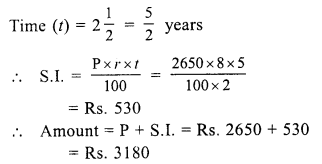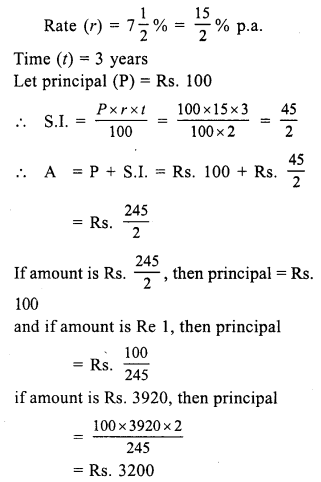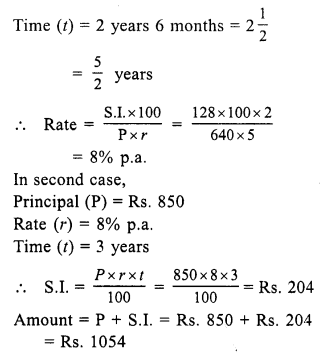# RS Aggarwal Class 7 Solutions Chapter 12 Simple Interest Ex 12A

In this chapter, we provide RS Aggarwal Solutions for Class 7 Chapter 12 Simple Interest Ex 12A for English medium students, Which will very helpful for every student in their exams. Students can download the latest RS Aggarwal Solutions for Class 7 Chapter 12 Simple Interest Ex 12A Maths pdf, free RS Aggarwal Solutions Class 7 Chapter 12 Simple Interest Ex 12A Maths book pdf download. Now you will get step by step solution to each question.

### RS Aggarwal Solutions for Class 7 Chapter 12 Simple Interest Ex 12A Download PDF

Question 1.
Solution:
Principal (P) = Rs. 6400
Rate (r) = 6% p.a.
Time (t) = 2 yearsQuestion 2.
Solution:
Principal (P) = Rs. 2650
Rate (r) = 8% p.a.Question 3.
Solution:
Principal (P) = Rs. 1500
Rate (r) = 12% p.a.Question 4.
Solution:
Principal (P) = Rs. 9600Question 5.
Solution:
Principal (P) = Rs. 5000
Rate (r) = 9% p.a.Find the time when :
Question 6.
Solution:
Principal (P) = Rs. 6400
S.I. = Rs. 1152
Rate (r) = 6% p.a.Question 7.
Solution:
Principal (P) = Rs. 9540
S.I. = Rs. 1908
Rate (r) = 8% p.a.Question 8.
Solution:
Amount (A) = Rs. 6450
Principal (P) = Rs. 5000
S.I. = A – P = Rs. (6450 – 5000) = Rs. 1450
Rate (r) = 12% p.a.Find the rate when :
Question 9.
Solution:
Principal (P) = Rs. 8250
S.I. = Rs. 1100
Time (t) = 2 yearsQuestion 10.
Solution:
Principal (P) = Rs. 5200
S.I. = Rs. 975Question 11.
Solution:
Principal (P) = Rs. 3560
Amount (A) = Rs. 4521.20
S.I. = A – P = Rs. 4521.20 – 3560 = Rs. 961.20
Time (t) = 3 yearsQuestion 12.
Solution:
Principal (P) = Rs. 6000
Rate (r) = 12% p.a.Question 13.
Solution:
Principal = Rs. 12600
Rate (A) = 15% p.a.
Time (t) = 3 yearsAmount = P + S.I. = Rs. 12600 + Rs. 5670 = Rs. 18270
Amount paid in cash = Rs. 7070
Balance = Rs. 18270 – 7070 = Rs. 11200
Price of goat = Rs. 11200

Question 14.
Solution:
S.I. = Rs. 829.50
Rate (r) = 10% p.a.
Time (t) = 3 yearsQuestion 15.
Solution:
Amount (A) = Rs. 3920Question 16.
Solution:
Amount = Rs. 4491
Let principal (P) = Rs. 100
Rate (r) =11% p.a.
Time (t) = 2 years 3 months = 214 = 94 yearsQuestion 17.
Solution:
Amount = Rs. 12122
Let principal (P) = Rs. 100
Rate (r) = 8% p.a.
Time (t) = 2 yearsQuestion 18.
Solution:
Amount (A) = Rs. 4734
Principal (P) = Rs. 3600
S.I. = A – P = Rs. 4734 – Rs. 3600 = Rs. 1134Question 19.
Solution:
In first case,
Amount (A) = Rs. 768
Principal (P) = Rs. 640
S.I. = A – P = Rs. 768 – 640 = Rs. 128Question 20.
Solution:
Principal (P) = Rs. 5600
Amount (A) = Rs. 6720
S.I. = A – P = Rs. 6720 – 5600 = Rs. 1120
Rate (r) = 8% p.a.Question 21.
Solution:
Let principal (P) = Rs. 100
then amount (A) = Rs. 100 x 85 = Rs. 160
S.I. = A – P = Rs. 160 – 100 = Rs. 60
Time (t) = 5 yearsQuestion 22.
Solution:
Amount in 3 years = Rs. 837
Amount in 2 years = Rs. 783
Difference = Rs. 837 – Rs. 783 = Rs. 54
Rs. 54 is interest for 1 year
Interest for 2 years = 2 x 54 = Rs. 108
Principal = Rs. 783 – 108 = Rs. 675Question 23.
Solution:
Amount in 5 years = Rs. 5475
Amount in 3 years = Rs. 4745
Interest for 2 years = Rs. 5475 – 4745 = Rs. 730Question 24.
Solution:
Total sum = Rs. 3000
Let first part = Rs. x
Then second part = Rs. (3000 – x)
Now, interest on first part at the rate ofQuestion 25.
Solution:
Total sum =Rs. 3600
Let first part = Rs. x
Then second part = Rs. (3600 – x)
Interest on first part for 1 year at 9% p.a.⇒ 9x + 10 (3600 – x) = 33300
⇒ 9x + 36000 – 10x = 33300
⇒ -x = 33300 – 36000
⇒ -x = – 2700
⇒ x = 2700
First part = Rs. 2700
and second part = Rs. 3600 – 2700 = Rs. 900

All Chapter RS Aggarwal Solutions For Class 7 Maths

—————————————————————————–

All Subject NCERT Exemplar Problems Solutions For Class 7

All Subject NCERT Solutions For Class 7

*************************************************

I think you got complete solutions for this chapter. If You have any queries regarding this chapter, please comment on the below section our subject teacher will answer you. We tried our best to give complete solutions so you got good marks in your exam.

If these solutions have helped you, you can also share rsaggarwalsolutions.in to your friends.